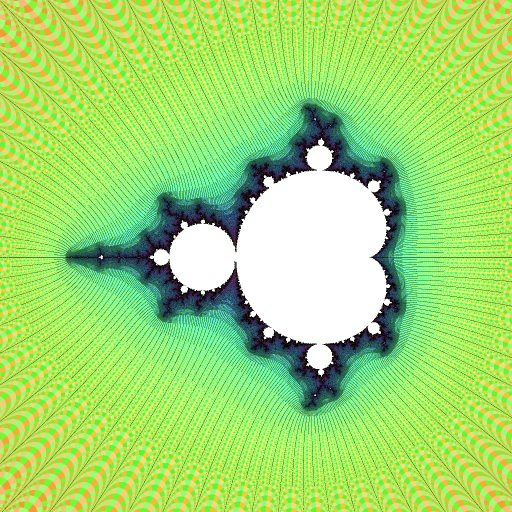# R2t Series

Robert P. Munafo, 2012 Apr 22.

The R2t Series is a series of mu-molecules on the real axis beginning with R2F(1/2B1)S and proceeding west towards the tip.

Each island in the series has a period one greater than the previous member of the series.

The series contains the smallest island of any given period. Here is a table of the first few:

 Period R2 name size rank Area Location (center and size) 3 R2F(1/2B1)S 1st 5.1023e-04 -1.759672 + 0i @ 0.067687 4 R2F(1/2B1)FSS 37th 1.4635e-06 -1.941076 + 0i @ 0.003627 5 R2F(1/2B1)FSS ~= 2177th 5.0830e-09 -1.985441 + 0i @ 0.000214 6 R2F(1/2B1)FSFSFSS ? ~= 1.9e-11 -1.99637834 + 0i @ 0.000013 7 R2F(1/2B1){FS}x4S ? ~= 7.4e-14 -1.9990964107298 + 0i @ 8.1e-7

This sequence includes the John Dewey Jones midget. Each successive island is located about 4 times closer to the tip at -2.0+0i. Each island is about 1/16 the size, and 1/256 the area of the one before it. Here is the island with period 27:leftmost period-27 island ` , ^. '- '\ \ ` . \ ,' . ,] ].`-.--.'_ /` r` _-` '-, `e `, \ ` \ . \',' \',`' [[..`/.`'.\/.-a- _-` , '- -, '- -,~,~,~,~ . -',`',\' ,[L'-.:\"/`"/\/`-_-` .,- `-, '`, '-,-,~,^,',^,",~,\'\',b:/\;/_.m--~://L~/'/`-`-`-' , ' , -,'\'.'\'\'\'\'.'e",)/@(.)/-/@@@@@ddF`/:-,L-\- -\ `-` -, . -,~ -,~,~,~,~,~/\-\(((C))aa\$\$@@@@@@@@m[/'\`c '-'-L,-`- ., `-,-.`.'-'-/-'-'\:((,;)))))a@c@Cb@@@@@"@@@@b))L/:[`..-d--:-- '-., , .` ,-'-'..v)))\\(~::d/F@d@@@@@@@[ .:@@@@@d@@b////-%;-:^ -.,-,`.`-,-'-'-\\`/@(a~_vv\c]bd@@@F*@*^^^` ^^^*T@@@@@@@//\"-%/-- _-.d-,-'-/-/,~~--\m@@@@mbb@@@@@@@@" `.@@@@@-L"`-L.^ -;--,-;-;d\-//::(7@@@@@@@@@@@@@@` 'Y\$@@C_-"-\-L ,-r\-':`['c)a'FCb@@@@@@^*^^^*@@` *@@@L_ar^^-- ^-'b.C\dbd@@@@@@@@@@@) '* .@@@@`@@@%.__ ---m/@@@@@@%@@@@@@C,_ -@@@F*****---- -%,\~7("YF@@F@@@@@@@@b, ..d '@@@@L___---- --.`-/~`]\/"\YYY@@@@@@@_meead@@e -d@@@L_,^^^_% :--:-:`d '-\\\\--(@@@@@@@@@@@@@@r_ .am@@@"_^`_^L- ^\-%,-`-\-.---///T*@F@"@@FY@@@@@@--_ ..,'@@@@@)\^_%--' '` ^\-'-',.,','/,~`(77^"--/~`T@@@@bm@mm__- -_emd@@@@@@F)/e\%-',; _,'' -\-_,','-'-',~)(@((....]]YY"@@@@@@@[, _d@@@@@@@@"/''',-:%-. ` _- -`,'-'.-.~.~.`/`;)))/--(C`Y@@@@@@@@b@@@@@d-^`]'[;-,-;--`- _,-` - -'.-_~.-.-.-.~."_~((:)@))~:(dC@@@@@@@@Y^[\]/-` / `-,-.` ` . - .- -`,`/'.'.'.`.`/`/`/^~(,)\/^Y@@@@@C['[:'`.-'-/-/-.`-, .-' .- .- './`/`,`,`/./.'a`/`'.`~^^^F*--('~/.-'\`.-.--`., - .- _~ .~ .~ /./.".~:`.`/:`/..`[[:'',-`',~'.`e~ -. ' , ., .-` ./` a~ ." ".'.-.`- /. ,. [\' c-',`'\`,` ~, -. -' -` -~ -` ~ ' ' - `' ' `' ' -- -' ` '- '- - -1.999999999999999178190346247658442 + 0i @ 7e-31, Nmax: 20000

The book Mm - Much Ado About Nothing - Vol. 1, (A.G. Davis Philip, Adam Robucci, Michael Frame & Kenelm Philip, LC catalog number 91-092943) discusses these islands. The last midget in their sequence is R2F(1/2B1){FS}x297S, with period 300, and its image is one of the deepest ever seen. To find the centers of these midgets, they discovered a general formula:

x = -2 + (6 (2j+1)2 π2) / 4N+1

where x is the real coordinate, N is the island's period, and j is a selector which should be zero.1 The formula gives a location a bit to the left of the actual island. Higher values of j locate other series of islands along the spike (try it!).

The Robucci formula above is not completely accurate for locating the leftmost island of period N. As N increases, the location given by the formula tends to drift gradually to the left of the desired point.

The following is much more accurate, and should properly center the view for N up to at least a few hundred:

center = -2 + 3π2/(2×4N) + 36.524N/16N - 100/16N
size = 200/16N

The first two terms are identical to the Robucci formula (with j=0); the next term (with coefficient 36.524) cancels the gradual "drift" in the Robucci formula. The last term is proportional to the size and therefore changing its coefficient will shift all islands the same amount in relation to the view.

This page was written in the "embarrassingly readable" markup language RHTF, and was last updated on 2020 Mar 26.s.11